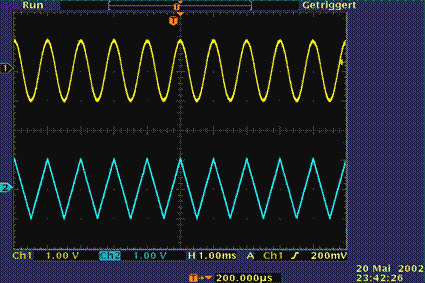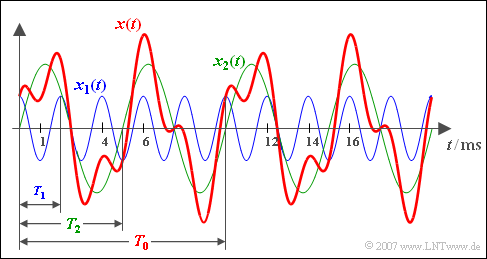# General Description

## # OVERVIEW OF THE SECOND MAIN CHAPTER #

In this chapter  periodic signals  are considered and described mathematically in the time and frequency domain.

This chapter contains in detail:

• Some basic terms like  "period duration", "basic frequency"  and  "circular frequency",
• the properties of a  "DC signal"  as a boundary case of a periodic signal,
• the definition and interpretation of the  "Dirac function",
• the spectral representation of a DC signal or a DC signal component,
• the time and frequency representation of  "harmonic oscillations", and finally
• the application of  "Fourier series"  for spectral analysis of periodic signals.

## Features and applications

Periodic signals are of great importance for communications engineering:

• They belong to the class of deterministic signals, whose time function can be specified in analytical form.
• Their signal path is thus known for all times  $t$  and can be clearly predicted for the future.
• They are therefore never information-carrying signals.

Nevertheless, periodic signals are often also required in communications engineering, for example

• for modulation and demodulation in carrier frequency systems,
• for synchronization and clock regeneration in digital systems,
• as test and verification signals during system implementation.Oscilloscope image of cosine and triangular signals

$\text{Example 1:}$  The oscilloscope image shows two typical representatives of periodic signals:

• above a cosine signal,
• below a triangular signal.

As can be seen from the displayed settings, the period duration of both signals is one millisecond and the amplitude one volt.

## Definition and parameters

Before we turn to the signal parameters of a periodic signal, the term  "periodicity"  shall be clearly defined:

$\text{Definition:}$  A  $\text{periodic signal}$  $x(t)$  is present if for all arbitrary values of  $t$  and all integer values of  $i$  with an appropriate  $T_{0}$  applies:

$$x(t+i\cdot T_{0}) = x(t).$$

This results in the following parameters:

• The  $\text{period duration}$  $T_{0}$  indicates the smallest possible value, which satisfies the above equation.
• The  $\text{basic frequency}$  $f_{0} = 1/T_{0}$  describes the number of periods per time unit (mostly per second).
• The unit  "1/s"  is also called  "Hz", named after the German physicist   Heinrich Hertz.
• The  $\text{basic circular frequency}$  $\omega_{0}$  represents the angular rotation per second, usually given in radians.
• In contrast to the basic frequency, the unit  "Hz"  is not common here, but  "1/s".  The following equation applies:
$$\omega_{0}=2\pi f_{0} = {2\pi}/{T_{0}}.$$

$\text{Example 2:}$  Here, a periodic time signal is shown:

• The period duration is  $T_{0} = 2.5 \ \rm ms$.
• From this the basic frequency   $f_0 = 400 \ \rm Hz$ is calculated.
• The basic circular frequency results to  $\omega_{0}=2513 \ \rm 1/s.$

## Resulting period duration

If a signal  $x(t)$  consists of the sum of two periodic signals  $x_{1}(t)$  and  $x_{2}(t)$  with the period durations  $T_{1}$  or   $T_{2}$, the resulting period duration of the sum signal is the smallest common multiple of  $T_{1}$  and  $T_{2}$.

• This statement applies independently of the amplitude and phase relations.
• On the other hand, if   $T_{1}$  and  $T_{2}$  don't have a rational common multiple  $($Example:   $T_{2} = \pi \cdot T_{1})$,
then the sum signal  $x(t)$  is in contrast to its two components  $x_{1}(t)$  and  $x_{2}(t)$  not periodic.

$\text{Example 3:}$  Here, a cosinusoidal signal  $x_{1}(t)$  with period duration  $T_{1} = 2\; {\rm ms}$  (blue signal course) is added with a sinusoidal signal  $x_{2}(t)$  with period duration  $T_{2} = 5\; {\rm ms}$  and twice the amplitude (green curve).Resulting period duration of the sum of cosine and sine signal
• The (red) sum signal  $x(t) = x_{1}(t) + x_{2}(t)$  then shows the resulting period duration  $T_{0} = 10\; {\rm ms}$   ⇒   basic frequency  $f_{0} = 100\; {\rm Hz}$.
• The frequency  $f_{0}$  itself is not contained in  $x(t)$  only integer multiples of it, namely
$f_{1} = 500\; {\rm Hz}$  and  $f_{2} = 200\; {\rm Hz}$.

With the interactive applet  Period Duration of Periodic Signals  the resulting period of two harmonic oscillations can be determined.